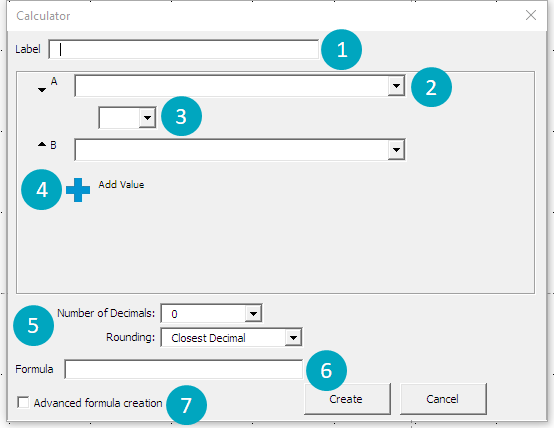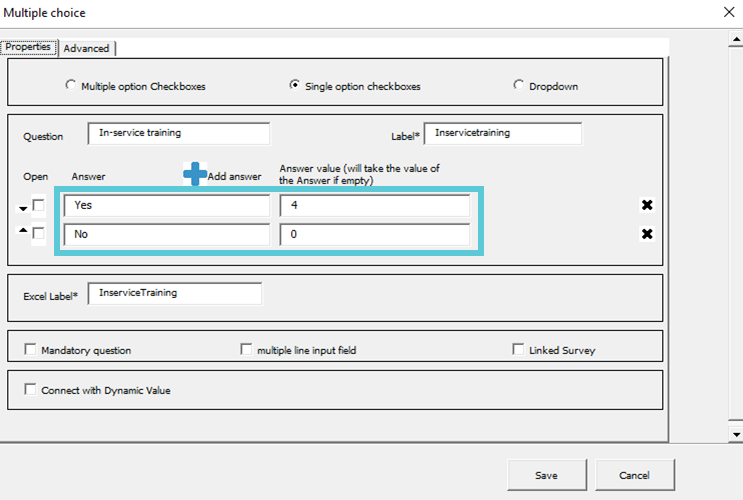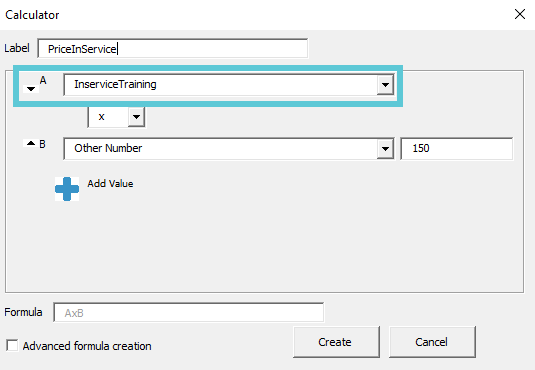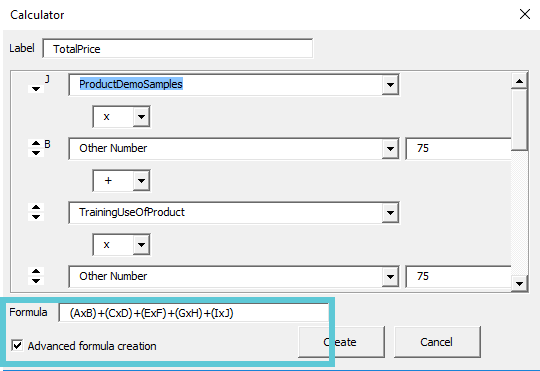# Working with the Calculate Result

Follow

With the 'Calculate Result'-function you can calculate with the result of other inserted d!nk elements.

After clicking on it in the menu bar:This pop-up will appear to create the needed calculation:1. Give your calculation a 'Display Label' to create a unique ID for this element.

2. Select the value you want to insert in your calculation. The result of another d!nk element (ex. multiple choice, hotzone,...) or choose 'Other value' to insert any number.

3. Choose the type of calculation (+, -, *, /) you need.

4. Add more options via the blue '+'

5. Define the number of decimals and rounding if you wish not to go with the default setting.
6. See and edit the formula of your calculation

7. If you select 'Advanced formula creation', you can add brackets in the 'Formula' to finetune your calculation, for instance to group the sums (A+B)x(C+D) instead of A+BxC+D or you can add a syntax, for example ^ in it to calculate a power: A^B.
All usable syntaxes can be found here: https://mathjs.org/docs/expressions/syntax.html

TUTORIAL WITH EXAMPLE

In this example we have built a list of services that the customer can choose from. Depending on the chosen services, the customer will get an overview of the total amount of working hours and the total price.

To calculate the price for each customer individually, we have used the 'Calculate Result'-function.

1. Insert d!nk elements

First we inserted the different services as multiple choice elements.The customer can choose 'yes' or 'no'. When the customer chooses 'yes' the value will be the total amount of hours for this service (in this case 4 hours). When the customer chooses 'no' the value will be '0'.2. Create calculation

In the summary we want to show the total price for each service the customer has chosen. If the customer selects "yes" he has to pay 4 hours * € 150. If the customer selects "no" he has to pay 0 hours * € 150.Value A is referring to the multiple choice element created in step 1.We made an extra calculation to know the total price of all chosen services.
Because the services have different prices/hour we used this formula: (service A * € 75 per hour) + (service B * € 75 per hour) + (service C * € 150 per hour).

In order to create this formula with parentheses we used the 'Advanced formula creation'.